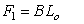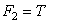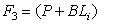# Soil Nail

The Soil Nail support type can be used to model soil nail slope reinforcement.

#### Notes:

• A Soil Nail is assumed to be fully bonded along its entire length
• The Soil Nail support type in Slide3 is equivalent to Grouted Tieback support with Bond Length = 100%
• The Soil Nail support type in Slide3 is differentiated from the Grouted Tieback for user convenience. However, the user should be aware that, as far as the Slide3 implementation is concerned, a Grouted Tieback with Bond Length = 100% would behave exactly the same as a Soil Nail, all other parameters being equal.
• A Soil Nail is NOT equivalent to the Grouted Tieback with Friction support type in Slide3.
• Soil Nails can also be used to model pile or micropile support by using the optional Shear or Compression options as described below.
Make sure that you have read the Overview of Support Implementation topic, for important information which applies to all support models in Slide3.

## Tensile Capacity

The Tensile Capacity entered for a Soil Nail, represents the maximum tensile capacity of an individual soil nail. This is the capacity of the soil nail itself (e.g. steel tensile capacity), independent of the plate capacity or the bond capacity. Units are Force.

## Plate Capacity

The Plate Capacity is the maximum load which can be sustained by the plate assembly which connects the soil nail to the slope. Units are Force.

## Shear Capacity

The Shear Capacity is optional and allows you to account for shear failure through the bolt cross-section (i.e. the force required to shear the bolt perpendicular to its axis). Units are Force. See below for implementation details.

## Compression Capacity

The Compression Capacity is optional and allows you to account for the failure of the bolt in compression. This can be useful for modelling compression piles, for example. Units are Force. See below for implementation details.

## Force Application

For Soil Nail support, the default method of Force Application = Active. See the Force Application topic for a discussion of the significance of Active and Passive support force application in Slide3.

## Force Orientation

For Soil Nail support, the orientation of the applied force is PARALLEL to the orientation of the soil nail, unless you are using the Shear Capacity option, in which case the force may be applied at some angle to the bolt axis, corresponding to the resultant of the shear and tensile components (see below for details).

## Pullout Strength

For a Soil Nail, the Pullout Strength is expressed as a Force per unit Length.

The length units, in this case, refer to the length along the soil nail. The Bond Strength determines the pullout and/or stripping force which can be generated by a soil nail.

## Material Dependent Pullout Strength

See the Grouted Tieback topic for details, as the procedure is the same.

## Implementation of Soil Nail Support in Slide3

Consider a soil nail which intersects a slip surface, as shown below (diagram is 2D but actual implementation is 3D).

Li = length of soil nail within sliding mass

Lo = length of soil nail embedded beyond slip surface

Soil Nail Parameters:

B = Bond Strength (force / unit length of soil nail)

T = Tensile Capacity (force)

P = Plate Capacity (force)

At any point along the length of the soil nail, there are 3 possible failure modes which are considered:

1. Pullout (force required to pull the length Lo of the nail out of the slope)
2. Tensile Failure (maximum axial capacity of the soil nail)
3. Stripping (slope failure occurs, but nail remains embedded in slope)

The maximum force which can be mobilized by each failure mode is given by the following equations:

Pullout:Eqn.1

Tensile:Eqn.2

Stripping:Eqn.3

At any point along the length of a soil nail, the force which is applied to the slip surface by the soil nail, is given by the MINIMUM of these three forces.

Applied Force = min (F1, F2, F3) Eqn.4

• In order for stripping to occur, the Plate Capacity must be exceeded. The Plate Capacity is included in the stripping force equation and added to the shear capacity along the length Li.
• If the Soil Nail Pullout Strength is specified as Material Dependent, then the Pullout Force and Stripping force, are determined by integrating along the lengths Lo and Li, to determine the force contributed by each segment of the soil nail which passes through different materials.

A typical Soil Nail Force diagram, which exhibits all three failure modes, is shown below. In this case, the Plate Capacity is less than the Tensile Capacity, and therefore "stripping" is a possible failure mode. If the Plate Capacity is greater than or equal to the Tensile Capacity, then stripping cannot occur, and the Soil Nail Force diagram will be determined only by the Tensile and Pullout failure modes.

## Implementation of Shear Capacity

If the Shear Capacity option is selected, an additional force vector perpendicular to the bolt direction, and opposite to the direction of failure, is added to the overall bolt capacity vector, determined by the methods described above. You’ll see this in Results mode when you show the support data. The support force at the base of the column is no longer parallel to the support but angled in a direction opposite to the slip direction. The Shear Capacity option may be useful if you expect that the support forces are not purely tensile, but may include a transverse shear component as well. This depends on the orientation of the support relative to the slip surface direction (diagram is 2D, the actual implementation is 3D).